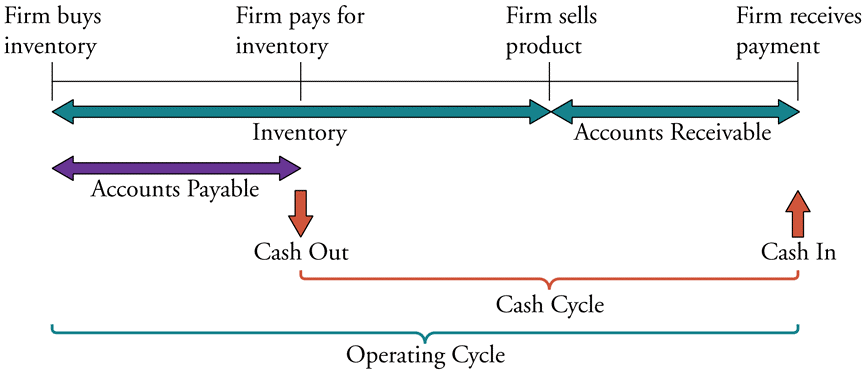# Fight Finance

#### CoursesTagsRandomAllRecentScores

 Portfolio Details Stock Expected return Standard deviation Correlation Beta Dollars invested A 0.2 0.4 0.12 0.5 40 B 0.3 0.8 1.5 80

What is the beta of the above portfolio?

You own an apartment which you rent out as an investment property.

What is the price of the apartment using discounted cash flow (DCF, same as NPV) valuation?

Assume that:

• You just signed a contract to rent the apartment out to a tenant for the next 12 months at $2,000 per month, payable in advance (at the start of the month, t=0). The tenant is just about to pay you the first$2,000 payment.
• The contract states that monthly rental payments are fixed for 12 months. After the contract ends, you plan to sign another contract but with rental payment increases of 3%. You intend to do this every year.
So rental payments will increase at the start of the 13th month (t=12) to be $2,060 (=2,000(1+0.03)), and then they will be constant for the next 12 months. Rental payments will increase again at the start of the 25th month (t=24) to be$2,121.80 (=2,000(1+0.03)2), and then they will be constant for the next 12 months until the next year, and so on.
• The required return of the apartment is 8.732% pa, given as an effective annual rate.
• Ignore all taxes, maintenance, real estate agent, council and strata fees, periods of vacancy and other costs. Assume that the apartment will last forever and so will the rental payments.

The 'initial margin', also known as the performance bond in a futures contract, is paid at the start when the futures contract is agreed to. or ?

There are many ways to calculate a firm's free cash flow (FFCF), also called cash flow from assets (CFFA).

One method is to use the following formulas to transform net income (NI) into FFCF including interest and depreciation tax shields:

$$FFCF=NI + Depr - CapEx -ΔNWC + IntExp$$

$$NI=(Rev - COGS - Depr - FC - IntExp).(1-t_c )$$

Another popular method is to use EBITDA rather than net income. EBITDA is defined as:

$$EBITDA=Rev - COGS - FC$$

One of the below formulas correctly calculates FFCF from EBITDA, including interest and depreciation tax shields, giving an identical answer to that above. Which formula is correct?

Acquirer firm plans to launch a takeover of Target firm. The deal is expected to create a present value of synergies totaling $2 million. A scrip offer will be made that pays the fair price for the target's shares plus 70% of the total synergy value.  Firms Involved in the Takeover Acquirer Target Assets ($m) 60 10 Debt ($m) 20 2 Share price ($) 10 8 Number of shares (m) 4 1

Ignore transaction costs and fees. Assume that the firms' debt and equity are fairly priced, and that each firms' debts' risk, yield and values remain constant. The acquisition is planned to occur immediately, so ignore the time value of money.

Calculate the merged firm's share price and total number of shares after the takeover has been completed.

The expression 'cash is king' emphasizes the importance of having enough cash to pay your short term debts to avoid bankruptcy. Which business decision is this expression most closely related to?

A semi-annual coupon bond has a yield of 3% pa. Which of the following statements about the yield is NOT correct? All rates are given to four decimal places.

An investor bought a bond for $100 (at t=0) and one year later it paid its annual coupon of$1 (at t=1). Just after the coupon was paid, the bond price was $100.50 (at t=1). Inflation over the past year (from t=0 to t=1) was 3% pa, given as an effective annual rate. Which of the following statements is NOT correct? The bond investment produced a: The below diagram shows a firm’s cash cycle.Which of the following statements about companies’ cash cycle is NOT correct? The arithmetic average and standard deviation of returns on the ASX200 accumulation index over the 24 years from 31 Dec 1992 to 31 Dec 2016 were calculated as follows: $$\bar{r}_\text{yearly} = \dfrac{ \displaystyle\sum\limits_{t=1992}^{24}{\left( \ln⁡ \left( \dfrac{P_{t+1}}{P_t} \right) \right)} }{T} = \text{AALGDR} =0.0949=9.49\% \text{ pa}$$ $$\sigma_\text{yearly} = \dfrac{ \displaystyle\sum\limits_{t=1992}^{24}{\left( \left( \ln⁡ \left( \dfrac{P_{t+1}}{P_t} \right) - \bar{r}_\text{yearly} \right)^2 \right)} }{T} = \text{SDLGDR} = 0.1692=16.92\text{ pp pa}$$ Assume that the log gross discrete returns are normally distributed and that the above estimates are true population statistics, not sample statistics, so there is no standard error in the sample mean or standard deviation estimates. Also assume that the standardised normal Z-statistic corresponding to a one-tail probability of 2.5% is exactly -1.96. Which of the following statements is NOT correct? If you invested$1m today in the ASX200, then over the next 4 years:

Copyright © 2014 Keith Woodward